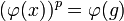# Powering-invariant over quotient-powering-invariant implies powering-invariant

(diff) ← Older revision | Latest revision (diff) | Newer revision → (diff)

## Statement

Suppose$G$ is a group and$H,K$ are subgroups of$G$ such that$H \le K \le G$,$H$ is normal in$G$, and the following conditions hold:

•$H$ is a quotient-powering-invariant subgroup of$G$.
•$K/H$ is a powering-invariant subgroup of$G/H$.

Then,$K$ is a powering-invariant subgroup of$G$.

## Proof

Given:$G$ is a group and$H,K$ are subgroups of$G$ such that$H \le K \le G$,$H$ is normal in$G$, and the following conditions hold:

•$H$ is a quotient-powering-invariant subgroup of$G$.
•$K/H$ is a powering-invariant subgroup of$G/H$.$p$ is a prime number such that$G$ is powered over$p$. An element$g \in K$.

To prove: There exists$x \in K$ such that$x^p = g$.

Proof: Let$\varphi:G \to G/H$ be the quotient map.

Step no. Assertion/construction Facts used Given data used Previous steps used Explanation
1 There exists$x \in G$ such that$x^p = g$.$G$ is$p$-powered. Given-direct.
2$G/H$ is$p$-powered.$H$ is quotient-powering-invariant in$G$,$G$ is$p$-powered. Given-direct.
3$(\varphi(x))^p = \varphi(g)$ and$\varphi(x)$ is the unique$p^{th}$ root of$\varphi(g)$ in$G/H$. Steps (1), (2) Applying the homomorphism$\varphi$ to Step (1) gives that$(\varphi(x))^p = \varphi(g)$. Since, by Step (2),$G/H$ is$p$-powered,$\varphi(x)$ must be the unique$p^{th}$ root.
4$\varphi(x) \in K/H$.$K/H$ is powering-invariant in$G/H$. Steps (2), (3) By Step (2),$G/H$ is$p$-powered, hence$K/H$ is$p$-powered from the given information. Thus, the unique$p^{th}$ root of$\varphi(g)$ in$G/H$, which equals$\varphi(x)$,(from Step (3)) must be in$K/H$.
5$x \in K$.$H \le K$ Step (4) Since$\varphi(x) \in K/H$,$x \in \varphi^{-1}(K/H)$, which is$K$ since$H \le K$.